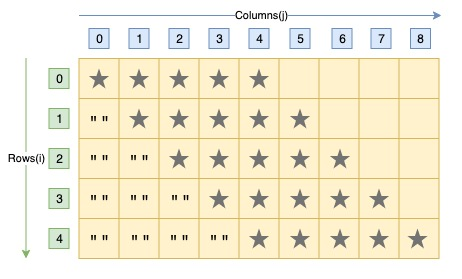# C Program to Print Rhombus Star Pattern

Here is a C program to print rhombus star pattern using for loop. For a rhombus star pattern of 6 rows. Program's output should be:#### Required Knowledge

Algorithm to print rhombus star pattern using loop
• We first take the number of rows(N) as input from user using scanf function.
• The index of rows and columns will start from 0.
• Each row of rhombus star pattern contains N star characters.
• Each iteration of outer for loop(from i = 0 to N-1) will print one row of pattern at a time.
• Each jth row, contains j space characters followed by N star characters.
• First inner for loop prints space characters whereas second inner for loop prints star characters.

Here is the matrix representation of the rhombus star pattern. The row numbers are represented by i whereas column numbers are represented by j.## C program to print rhombus star pattern

```#include <stdio.h>

int main() {
int i, j, rows;

printf("Enter the number of rows\n");
scanf("%d", &rows);

for (i = 0; i < rows; i++) {
/* Print spaces before stars in a row */
for (j = 0; j < i; j++) {
printf(" ");
}
/* Print rows stars after spaces in a row */
for (j = 0; j < rows; j++) {
printf("*");
}
printf("\n");
}
return 0;
}
```
Output
```Enter the number of rows
6
******
******
******
******
******
******
```

Related Topics
 C program hollow diamond star pattern C program pyramid star pattern C program rectangle star pattern C program hollow pyramid star pattern C program square star pattern C program hollow rectangle pattern C program mirrored right triangle star pattern C program prime number triangle pattern C program multiplication table triangle pattern List of all C pattern programs